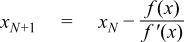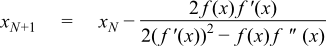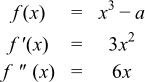Boost C++ Libraries

...one of the most highly regarded and expertly designed C++ library projects in the world.

This is the documentation for an old version of boost. Click here for the latest Boost documentation.

Root Finding With Derivatives: Newton-Raphson, Halley & Schroeder

Synopsis

#include <boost/math/tools/roots.hpp>

namespace boost{ namespace math{
namespace tools{

template <class F, class T>
T newton_raphson_iterate(F f, T guess, T min, T max, int digits);

template <class F, class T>
T newton_raphson_iterate(F f, T guess, T min, T max, int digits, boost::uintmax_t& max_iter);

template <class F, class T>
T halley_iterate(F f, T guess, T min, T max, int digits);

template <class F, class T>
T halley_iterate(F f, T guess, T min, T max, int digits, boost::uintmax_t& max_iter);

template <class F, class T>
T schroeder_iterate(F f, T guess, T min, T max, int digits);

template <class F, class T>
T schroeder_iterate(F f, T guess, T min, T max, int digits, boost::uintmax_t& max_iter);

}}} // namespaces
Description

These functions all perform iterative root finding using derivatives:

The functions all take the same parameters:

Parameters of the root finding functions

F f

Type F must be a callable function object that accepts one parameter and returns a boost::math::tuple:

For the second order iterative methods (Newton Raphson) the boost::math::tuple should have two elements containing the evaluation of the function and its first derivative.

For the third order methods (Halley and Schroeder) the boost::math::tuple should have three elements containing the evaluation of the function and its first and second derivatives.

T guess

The initial starting value. A good guess is crucial to quick convergence!

T min

The minimum possible value for the result, this is used as an initial lower bracket.

T max

The maximum possible value for the result, this is used as an initial upper bracket.

int digits

The desired number of binary digits.

uintmax_t max_iter

An optional maximum number of iterations to perform.

When using these functions you should note that:

• Default max_iter = (std::numeric_limits<boost::uintmax_t>::max)() is effectively 'iterate for ever'!.
• They may be very sensitive to the initial guess, typically they converge very rapidly if the initial guess has two or three decimal digits correct. However convergence can be no better than bisection, or in some rare cases, even worse than bisection if the initial guess is a long way from the correct value and the derivatives are close to zero.
• These functions include special cases to handle zero first (and second where appropriate) derivatives, and fall back to bisection in this case. However, it is helpful if functor F is defined to return an arbitrarily small value of the correct sign rather than zero.
• If the derivative at the current best guess for the result is infinite (or very close to being infinite) then these functions may terminate prematurely. A large first derivative leads to a very small next step, triggering the termination condition. Derivative based iteration may not be appropriate in such cases.
• If the function is 'Really Well Behaved' (monotonic and has only one root) the bracket bounds min and max may as well be set to the widest limits like zero and numeric_limits<T>::max().
• But if the function more complex and may have more than one root or a pole, the choice of bounds is protection against jumping out to seek the 'wrong' root.
• These functions fall back to bisection if the next computed step would take the next value out of bounds. The bounds are updated after each step to ensure this leads to convergence. However, a good initial guess backed up by asymptotically-tight bounds will improve performance no end - rather than relying on bisection.
• The value of digits is crucial to good performance of these functions, if it is set too high then at best you will get one extra (unnecessary) iteration, and at worst the last few steps will proceed by bisection. Remember that the returned value can never be more accurate than f(x) can be evaluated, and that if f(x) suffers from cancellation errors as it tends to zero then the computed steps will be effectively random. The value of digits should be set so that iteration terminates before this point: remember that for second and third order methods the number of correct digits in the result is increasing quite substantially with each iteration, digits should be set by experiment so that the final iteration just takes the next value into the zone where f(x) becomes inaccurate.
• To get the binary digits of accuracy, use policies::get_max_root_iterations<Policy>()).
• If you need some diagnostic output to see what is going on, you can #define BOOST_MATH_INSTRUMENT before the #include <boost/math/tools/roots.hpp>, and also ensure that display of all the possibly significant digits with cout.precision(std::numeric_limits<double>::max_digits10): but be warned, this may produce copious output!
• Finally: you may well be able to do better than these functions by hand-coding the heuristics used so that they are tailored to a specific function. You may also be able to compute the ratio of derivatives used by these methods more efficiently than computing the derivatives themselves. As ever, algebraic simplification can be a big win.
Newton Raphson Method

Given an initial guess x0 the subsequent values are computed using:Out of bounds steps revert to bisection of the current bounds.

Under ideal conditions, the number of correct digits doubles with each iteration.

Halley's Method

Given an initial guess x0 the subsequent values are computed using:Over-compensation by the second derivative (one which would proceed in the wrong direction) causes the method to revert to a Newton-Raphson step.

Out of bounds steps revert to bisection of the current bounds.

Under ideal conditions, the number of correct digits trebles with each iteration.

Schroeder's Method

Given an initial guess x0 the subsequent values are computed using:Over-compensation by the second derivative (one which would proceed in the wrong direction) causes the method to revert to a Newton-Raphson step. Likewise a Newton step is used whenever that Newton step would change the next value by more than 10%.

Out of bounds steps revert to bisection of the current bounds.

Under ideal conditions, the number of correct digits trebles with each iteration.

Example

Let's suppose we want to find the cube root of a number: the equation we want to solve along with its derivatives are:To begin with lets solve the problem using Newton-Raphson iterations, we'll begin by defining a function object (functor) that returns the evaluation of the function to solve, along with its first derivative f'(x):

template <class T>
struct cbrt_functor
{
cbrt_functor(T const& target) : a(target)
{ // Constructor stores value to be 'cube-rooted'.
}
boost::math::tuple<T, T> operator()(T const& z)
{ // z is estimate so far.
return boost::math::make_tuple(
z*z*z - a, // return both f(x)
3 * z*z);  // and f'(x)
}
private:
T a; // to be 'cube-rooted'.
};

Implementing the cube root is fairly trivial now, the hardest part is finding a good approximation to begin with: in this case we'll just divide the exponent by three:

template <class T>
T cbrt(T z)
{
using namespace std; // for frexp, ldexp, numeric_limits.
using namespace boost::math::tools;

int exp;
frexp(z, &exp); // Get exponent of z (ignore mantissa).
T min = ldexp(0.5, exp/3);
T max = ldexp(2.0, exp/3);
T guess = ldexp(1.0, exp/3); // Rough guess is to divide the exponent by three.
int digits = std::numeric_limits<T>::digits; // Maximum possible binary digits accuracy for type T.
return newton_raphson_iterate(detail::cbrt_functor<T>(z), guess, min, max, digits);
}

Using the test data in libs/math/test/cbrt_test.cpp this found the cube root exact to the last digit in every case, and in no more than 6 iterations at double precision. However, you will note that a high precision was used in this example, exactly what was warned against earlier on in these docs! In this particular case it is possible to compute f(x) exactly and without undue cancellation error, so a high limit is not too much of an issue. However, reducing the limit to std::numeric_limits<T>::digits * 2 / 3 gave full precision in all but one of the test cases (and that one was out by just one bit). The maximum number of iterations remained 6, but in most cases was reduced by one.

Note also that the above code omits a probably optimization by computing z², and reusing it, omits error handling, and does not handle negative values of z correctly. (These are left as an exercise for the reader!)

The boost::math::cbrt function also includes these and other improvements.

Now let's adapt the functor slightly to return the second derivative as well:

template <class T>
struct cbrt_functor
{
cbrt_functor(T const& target) : a(target){}
boost::math::tuple<T, T, T> operator()(T const& z)
{
return boost::math::make_tuple(
z*z*z - a,
3 * z*z,
6 * z);
}
private:
T a;
};

And then adapt the cbrt function to use Halley iterations:

template <class T>
T cbrt(T z)
{
using namespace std;
using namespace boost::math::tools;

int exp;
frexp(z, &exp);
T min = ldexp(0.5, exp/3);
T max = ldexp(2.0, exp/3);
T guess = ldexp(1.0, exp/3);
int digits = std::numeric_limits<T>::digits / 2;
return halley_iterate(detail::cbrt_functor<T>(z), guess, min, max, digits);
}

Note that the iterations are set to stop at just one-half of full precision, and yet, even so, not one of the test cases had a single bit wrong. What's more, the maximum number of iterations was now just 4.

Just to complete the picture, we could have called schroeder_iterate in the last example: and in fact it makes no difference to the accuracy or number of iterations in this particular case. However, the relative performance of these two methods may vary depending upon the nature of f(x), and the accuracy to which the initial guess can be computed. There appear to be no generalisations that can be made except "try them and see".

Finally, had we called cbrt with NTL::RR set to 1000 bit precision, then full precision can be obtained with just 7 iterations. To put that in perspective, an increase in precision by a factor of 20, has less than doubled the number of iterations. That just goes to emphasise that most of the iterations are used up getting the first few digits correct: after that these methods can churn out further digits with remarkable efficiency.

Or to put it another way: nothing beats a really good initial guess!